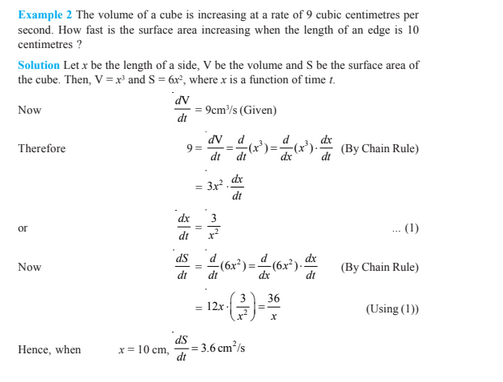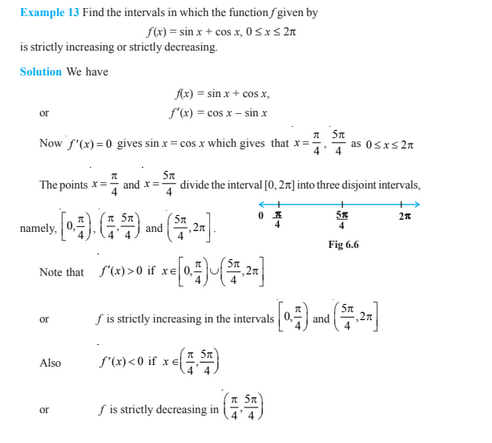# Application Of Derivatives For Class 12

In applications of derivatives class 12 chapter 6, we will study different applications of derivatives in various fields like Science, Engineering, and many other fields. In chapter 6, we are going to learn how to determine the rate of change of quantity, finding the equations of tangents, finding turning points on the graphs for various functions, maxima and minima and so on. Let us discuss some important concepts involved in the application of derivatives class 12 in detail.

## Application of Derivatives Class 12 Concepts

The topic and subtopics covered in applications of derivatives class 12 chapter 6 are:

• Introduction
• Rate of Change of Quantities
• Increasing and Decreasing Functions
• Tangents and Normals
• Approximations
• Maxima and Minima
• Maximum and Minimum Values of a Function in a Closed Interval

### Application of Derivatives Class 12 Notes

Let us discuss the important concepts involved in applications of derivatives class 12 with examples.

Rate of change of quantity-

Consider a function y = f(x), the rate of change of a function is defined as-

dy/dx = f'(x)

Further, if two variables x and y are varying to another variable, say if x = f(t), and y = g(t), then using Chain Rule, we have:

dy/dx = (dy/dt)/(dx/dt)

where dx/dt is not equal to 0.

Increasing and Decreasing Functions:

Consider a function f, continuous in [a,b] and differentiable on the open interval (a,b), then

(i) f is increasing in [a,b] if f'(x)>0 for each x in (a,b)

(ii) f is decreasing in [a,b] if f'(x)< 0 for each x in (a,b)

(iii) f is constant function in [a,b], if  f'(x) = 0 for each x in (a,b)

### Applications of Derivatives Class 12 ExamplesStay tuned with BYJU’S – The Learning App for more class 12 Maths concepts also read related articles to learn the topic with ease.

 Read More Class 12 concepts Determinants For Class 12 Differential Equations For Class 12 Integrals For Class 12 Linear Programming For Class 12